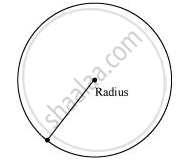# Give a definition for radius of a circle. Are there other terms that need to be defined first? What are they, and how might you define them? - Mathematics

Give a definition for radius of a circle. Are there other terms that need to be defined first? What are they, and how might you define them?

#### Solution

It is the distance between the centres of a circle to any point lying on the circle.

To define the radius of a circle, we must know about point and circle.Concept: Euclid’S Definitions, Axioms and Postulates
Is there an error in this question or solution?
Chapter 5: Introduction to Euclid's Geometry - Exercise 5.1 [Page 85]

#### APPEARS IN

NCERT Class 9 Maths
Chapter 5 Introduction to Euclid's Geometry
Exercise 5.1 | Q 2.4 | Page 85

Share